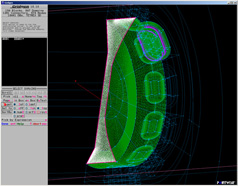# Computational Fluid Dynamics – What is CFD?## A Short Explanation of CFD

Computational Fluid Dynamics (CFD) is an engineering software approach that uses numerical techniques to simulate fluid flow. The purpose is to understand from a design and analysis perspective how fluid dynamics affects the operation or performance of a hardware component or system. For example, one can use CFD to compute the air flow around a wing to study lift and drag, to analyze the heat transfer rate on a high speed missile to develop a better thermal protection system, or to visualize the flow of solid particles in a rocket motor to predict slag accumulation and nozzle erosion. CFD is often referred to as a “numerical wind tunnel” or a “numerical test bed” because of its ability to simulate complex fluid flows that are often difficult to replicate in experiments.

There are generally 3 steps in obtaining a CFD solution:

1. Discretize the surface geometry and the physical space around it (i.e., generate a computational mesh consisting of discrete volumes that fill the space).
2. Numerically solve the governing equations of fluid dynamics for the pressure, temperature, velocity, etc. within each finite volume (this includes the bounding surfaces such as the missile body).
3. Use a post-processing tool to visualize the solution and to extract useful engineering information from it (e.g., lift, heat transfer rate, particle trajectories, etc.).

The process is conceptually very simple, but the details are often quite complex (see Computational Fluid Dynamics on Wikipedia for a flavor of the additional technical detail). In addition, it is generally good practice to anchor the CFD model with experimental data to understand the limits of applicability and the uncertainties of the predictions before applying simulation results to real systems (but note that this is not always possible to the extent desired). Once the CFD model is ready, geometry and flow conditions can be altered to give the analyst a powerful tool to examine the solution over a wide range of parameters for designing and analyzing new hardware.